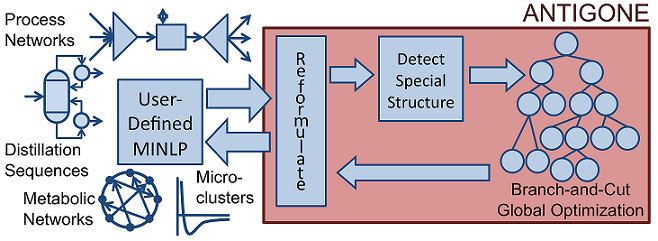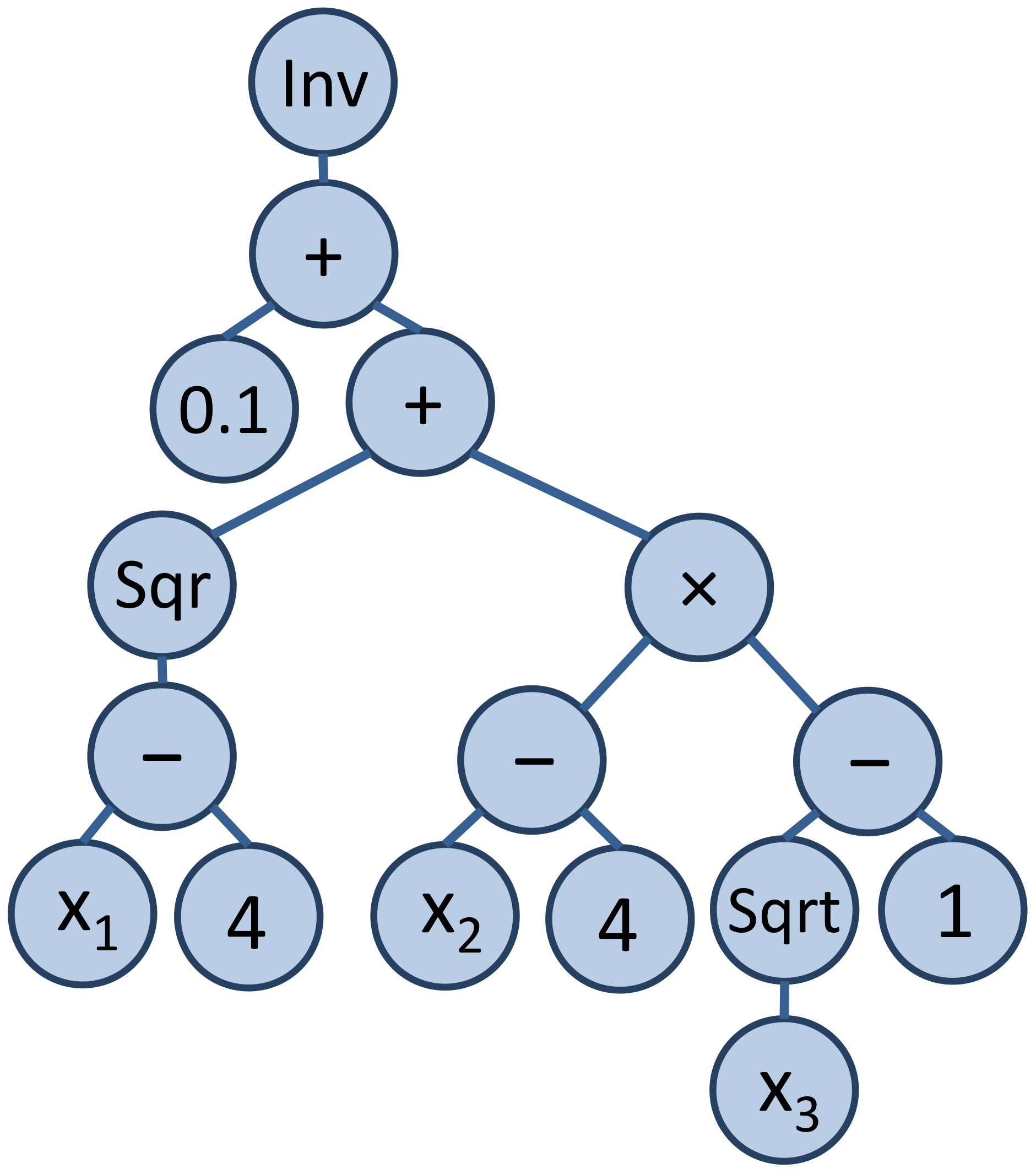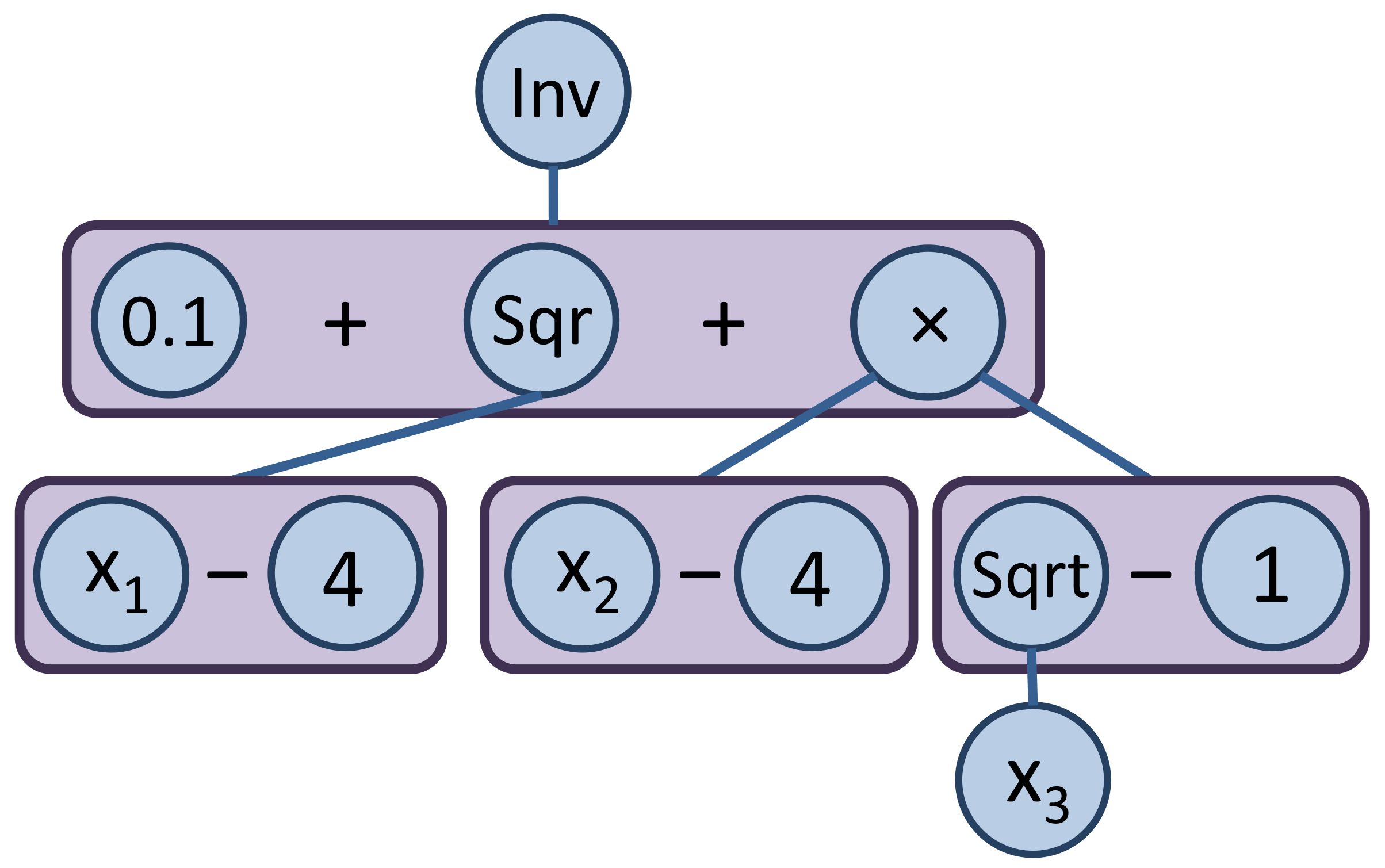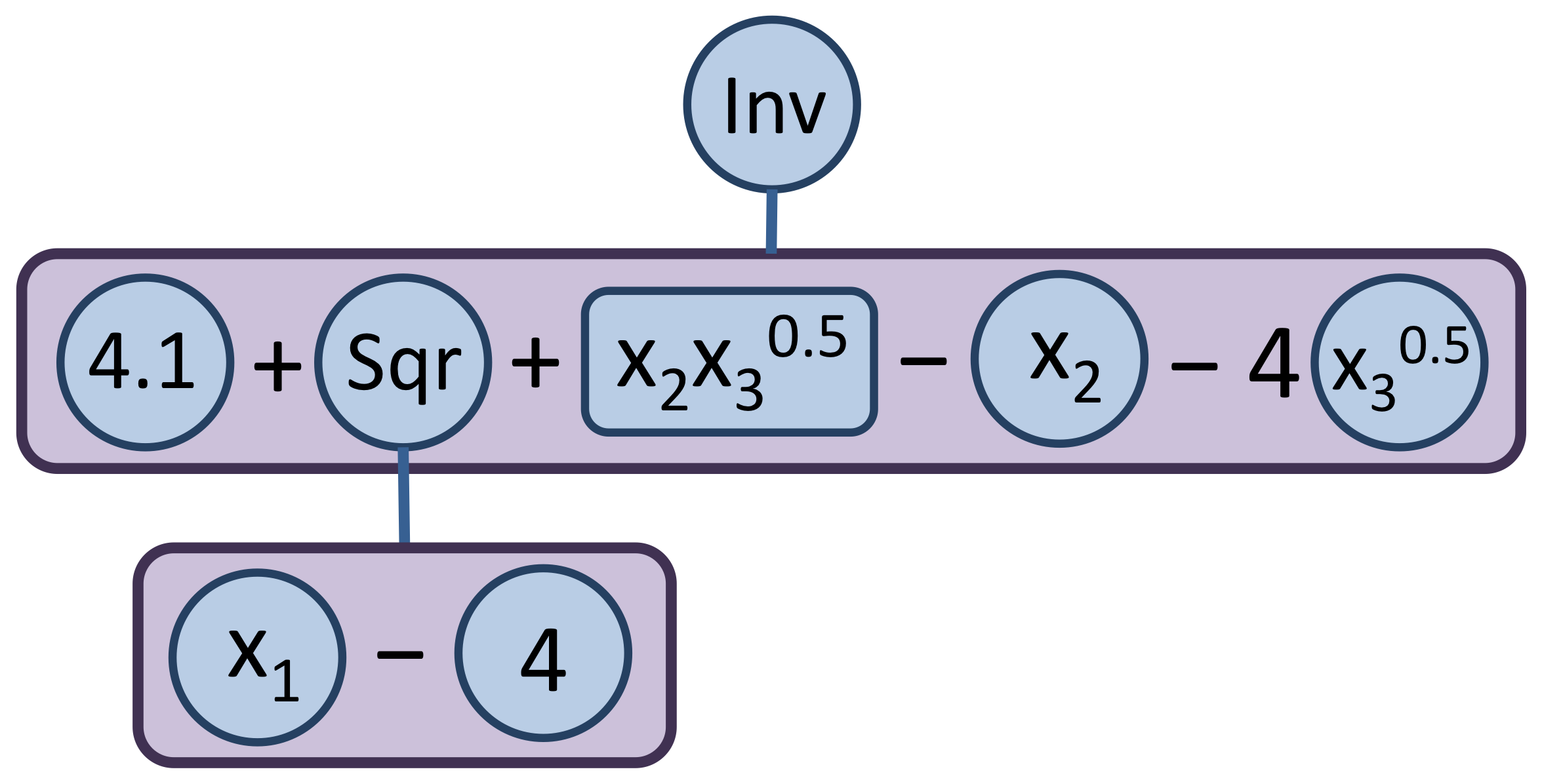ANTIGONE

Date
16 April 2013
Version
ANTIGONE 1.0

# Introduction

ANTIGONE, Algorithms for coNTinuous / Integer Global Optimization of Nonlinear Equations, is a deterministic general mixed-integer nonlinear global optimization framework [180, 181, 182, 183, 186] .

MINLP is defined:

$\begin{array}{rl} \tag{MINLP} \min \; & \phantom{b_m^{\mathrm{LO}} \leq {}} f_0(\boldsymbol{x}, \, \boldsymbol{y}, \, \boldsymbol{z}) \\[8pt] \text{s.t. } & b_m^{\mathrm{LO}} \leq f_m(\boldsymbol{x}, \, \boldsymbol{y}, \, \boldsymbol{z}) \leq b_m^{\mathrm{UP}} \quad \forall \; m \in \{ 1, \, \ldots, \, M \} \\[10pt] & \boldsymbol{x} \in \mathbb{R}^{C}; \; \boldsymbol{y} \in \left\{ 0, \, 1 \right\}^{B}; \; \boldsymbol{z} \in \mathbb{Z}^{I} \\ \end{array}$

where $$C$$, $$B$$, $$I$$, and $$M$$ represent the number of continuous variables, binary variables, integer variables, and constraints, respectively. Parameters vectors $$\boldsymbol{b_m^{\mathrm{LO}}}$$ and $$\boldsymbol{b_m^{\mathrm{UP}}}$$ bound the constraints. We assume that it is possible to infer finite bounds $$\left[ x_i^L, \, x_i^U \right]$$ on the variables participating in nonlinear terms $$f_m$$ and that the image of $$f_m$$ is finite on $$\boldsymbol{x}$$. Typical expressions for $$f_0(\boldsymbol{x}, \, \boldsymbol{y}, \, \boldsymbol{z})$$ and $$f_m(\boldsymbol{x}, \, \boldsymbol{y}, \, \boldsymbol{z})$$ are:

$\begin{split} f_m(\boldsymbol{x}, \, \boldsymbol{y}, \, \boldsymbol{z}) = c_m & + a_m^T \, \left[ \boldsymbol{x}; \; \boldsymbol{y}; \; \boldsymbol{z} \right] + \left[ \boldsymbol{x}; \; \boldsymbol{y}; \; \boldsymbol{z} \right]^T \, Q_m \, \left[ \boldsymbol{x}; \; \boldsymbol{y}; \; \boldsymbol{z} \right] \\ & + \sum\limits_{s = 1}^{S_m} c_{s_m} \cdot \prod\limits_{c = 1}^C x_c^{p_{s_m, \, c}} + \sum\limits_{e = 1}^{E_m} c_{e_m} \cdot e^x + \sum\limits_{\ell = 1}^{L_m} c_{\ell_m} \cdot \log x \end{split}$

where the powers $$p_{s_m, \, c}$$ are constant reals; $$c_m, \, a_m, \, Q_m, \, c_{s_m}, \, c_{e_m}, \, c_{\ell_m}$$ are constant coefficients; $$S_m$$, $$E_m$$, $$L_m$$ are the number of signomial, exponential, and logarithmic terms, respectively.

As illustrated in the following figure, ANTIGONE responds dynamically to exploit special structure within (MINLP). ANTIGONE falls broadly into the category of branch-and-bound global optimization because it: generates and solves convex relaxations of the nonconvex MINLP that rigorously bound the global solution; finds feasible solutions via local optimization; divides and conquers the feasible set to generate a sequence of convex relaxations converging to the global optimum [93, 90] .Given an MINLP optimization problem, ANTIGONE reformulates the model, detects special structure in the reformulated MINLP, solves the optimization problem, and returns the model with respect to the original problem variables

## Licensing and software requirements

Using GAMS/ANTIGONE requires

3. a CONOPT or SNOPT license.

ANTIGONE performs equivalently to GAMS/GloMIQO in the case where all nonlinearities in (MINLP) are quadratic; an ANTIGONE license includes the use of GloMIQO.

## Running GAMS/ANTIGONE

GAMS/ANTIGONE solves: NLP; MINLP; RMINLP; QCP; MIQCP; RMIQCP; CNS. If GAMS/ANTIGONE is not the default solver for these models, it can be called using the following command before the solve statement:

option nlp=antigone, minlp=antigone, rminlp=antigone;


# GAMS/ANTIGONE Output

The log output shown below is generated using the MINLP model cecil_13 from the MINLPLib.

-------------------------------------------------------------------------------
ANTIGONE: Algorithms for coNTinuous/Integer Global Optimization; Version 1.0

Ruth Misener and Christodoulos A. Floudas

Computer-Aided Systems Laboratory (CASL)
Department of Chemical & Biological Engineering; Princeton University
-------------------------------------------------------------------------------

Before Pre-processing:
840 Variables
660 Continuous
180 Binary
929 Equations

After Pre-processing:
520 Variables
418 Continuous
102 Binary
499 Equations
291 Linear
208 Nonconvex nonlinear
232 Nonlinear Terms
232 Signomial
730 Possible Reformulation Linearization Technique (RLT) equations
34 RLT Equations Added Outright to Formulation

Constituent Libraries:
CPLEX  Solving relaxations
CONOPT Finding feasible points
Boost  Bounding Intervals

-------------------------------------------------------------------------------
Time (s) Nodes explored Nodes remaining Best possible   Best found Relative Gap
-------------------------------------------------------------------------------

65              1               1    -1.158e+05   -1.157e+05   +1.032e-03
134              1               1    -1.157e+05   -1.157e+05   +4.337e-04
202              1               1    -1.157e+05   -1.157e+05   +4.334e-04
258              1               1    -1.157e+05   -1.157e+05   +4.140e-06
Solving MILP relaxation at tree level 0 ----------------------------------
341              1               1    -1.157e+05   -1.157e+05   +4.091e-06
413              1               1    -1.157e+05   -1.157e+05   +4.011e-06
Solving MILP relaxation at tree level 0 ----------------------------------
483              1               1    -1.157e+05   -1.157e+05   +4.001e-06
571              1               1    -1.157e+05   -1.157e+05   +3.959e-06
Solving MILP relaxation at tree level 0 ----------------------------------
640              1               1    -1.157e+05   -1.157e+05   +3.880e-06
Solving MILP relaxation at tree level 0 ----------------------------------
702              1               0    -1.157e+05   -1.157e+05   +1.000e-06

-------------------------------------------------------------------------------
Termination Status : Global minimum
Best Feasible Point: -1.156565e+05
Best Possible Point: -1.156566e+05
Relative Gap: +1.000000e-06
Algorithm analysis :

0 Nodes explored
0 Nodes remaining

0 Maximum tree depth

183 Cutting Planes (183 Globally Valid)
183 Signomial

702.38 Total time (CPU s)
0.07 Pre-processing
698.56 Solving MILP relaxations
0.95 Searching for feasible solutions
2.79 Variable bounds tightening
2.31 OBBT
1.48 FBBT (0.13 EC; 0.92 RLT; 0.00 Factoring)
0.00 Branching
0.00 Reliability branching
-------------------------------------------------------------------------------


# Summary of ANTIGONE Options

## General Options

Option Description Default
abs_opt_tol absolute stopping tolerance GAMS optca
dumpsolutions name of solutions index gdx file for writing alternate solutions
max_number_nodes node limit GAMS nodlim
max_time resource limit GAMS reslim
rel_opt_tol relative stopping tolerance GAMS optcr
trydual call CONOPT or SNOPT to produce duals 5

## Options for Solving the MILP Relaxations

Option Description Default
cplex_optfile read a secondary GAMS/CPLEX options file that will be applied to every LP and MILP subsolve
cut_generation_epsilon absolute violation threshold for separating hyperplanes 1e-4
nominal_time_limit nominal time limit for solving MILP subproblems 100
populate_solution_pool emphasis on generating starting points 3

## Options for Finding Feasible Solutions

Option Description Default
conopt_optfile read a secondary GAMS/CONOPT options file that will be applied to every NLP subsolve
feas_soln_time_limit time limit (s) for an NLP solve 30
feas_tolerance absolute feasibility tolerance 1e-6
nlp_solver use CONOPT or SNOPT to find feasible solutions conopt

## Options for Branching

Option Description Default
branching_bounds_push_away branch a minimum fraction away from the variable bounds 0.1
branching_weight branch on a convex combination of midpoint and solution 0.25
num_reliability_tests number of strong branching initialization tests 8
reliability_branching heuristic choice for building reliable pseudocosts error
reliability_branching_mu score parameter for building reliability 0.15
use_reliability_branching use reliability branching? 1

## Options for Bounding

Option Description Default
fbbt_improvement_bound bounds reduction improvement threshold needed to exit FBBT loop 0.999
max_fbbt_iterations maximum number of FBBT iterations 50
max_obbt_iterations maximum number of OBBT iterations 30
max_time_each_obbt time limit (s) for each OBBT LP 10
obbt_improvement_bound bounds reduction improvement threshold 0.95
use_obbt use optimality-based bounds tightening? 1

## Options for Logging to the Console

Option Description Default
logging_freq how often should we log progress to the console? 5
logging_level logging information level -1
print_options print the option parameter choices used in a single run? 1

## Options for Addressing Special Structure

Option Description Default
adaptive_add_rlt use the dynamic approach to adaptively determine deep RLT cuts? 1
adaptive_add_rlt_tree_depth tree depth for heuristic that adaptively determines deep RLT cuts 3
add_bilinear_terms allow addition of nonconvex bilinear terms to generate deep RLT cuts 1
convexity_cuts derive convexity-based separating cuts for multivariable terms? 1
dominant_ec_only add only the low-dimension edge-concave aggregations introducing dominant cuts into relaxations? 1
eigenvector_projections use eigenvector projections as additional cuts? 1
eigenvector_projection_partitioning allow partitioning on eigenvector projections? 1
low_dim_edge_concave_agg use low-dimension edge-concave aggregations? 1
max_partitioned_quantities number of partitioned quantities 0
max_rlt_cuts maximum number of violated RLT cuts to add before resolving the relaxation? 100
naive_add_ec naively integrate all low-dimension edge-concave aggregations into relaxations? 0
naive_add_rlt naively add all RLT cuts to the relaxations? 0
number_of_partitions how many partitions per variable? 1
partitioning_scheme Partitioning scheme can be linear or logarithmic linear
piecewise_linear_partitions use piecewise-linear partitioning? 0
rlt find RLT variable/equation and equation/equation pairs? 1
use_alpha_bb apply globally-valid alphaBB cuts to tighten a node relaxation 1
use_edge_concave_dynamic apply locally-valid edge-concave cuts to tighten a node relaxation 1

# Detailed Descriptions of ANTIGONE Options

abs_opt_tol (real): absolute stopping tolerance

Default: GAMS optca

In the first few levels of the branch-and-bound tree, query the RLT equations after solving an initial relaxation. Add violated equations to the relaxation and resolve. Track the most commonly-violated equations and include those cuts in later nodes.

Default: 1

To the specified tree depth, solve the relaxation of a node twice if RLT equations are violated. After this depth, automatically add the most commonly violated cuts to the solution of each node

Range: {1, ..., 100}

Default: 3

add_bilinear_terms (boolean): allow addition of nonconvex bilinear terms to generate deep RLT cuts

Default: 1

branching_bounds_push_away (real): branch a minimum fraction away from the variable bounds

Range: [0, 0.5]

Default: 0.1

branching_weight (real): branch on a convex combination of midpoint and solution

The branching weight specifies the emphasis on the midpoint of a variable, so larger branching weights imply branching closer to the center of a variable range.

Range: [0, 1]

Default: 0.25

conopt_optfile (string): read a secondary GAMS/CONOPT options file that will be applied to every NLP subsolve

Gain direct access to the GAMS/CONOPT options. The value of the string should match the name of the GAMS/CONOPT options file.

convexity_cuts (boolean): derive convexity-based separating cuts for multivariable terms?

Default: 1

cplex_optfile (string): read a secondary GAMS/CPLEX options file that will be applied to every LP and MILP subsolve

Gain direct access to the GAMS/CPLEX options. Specifying an options file allows, for example, the possibility of running the CPLEX subsolver with multiple threads. The value of the string should match the name of the GAMS/CPLEX options file.

cut_generation_epsilon (real): absolute violation threshold for separating hyperplanes

Absolute violation threshold to generate separating hyperplanes for convex multivariable terms

Range: [1e-7, 10]

Default: 1e-4

dominant_ec_only (boolean): add only the low-dimension edge-concave aggregations introducing dominant cuts into relaxations?

Default: 1

dumpsolutions (string): name of solutions index gdx file for writing alternate solutions

The GDX file specified by this option will contain a set call index that contains the names of GDX files with the individual solutions. For details see example model dumpsol in the GAMS Test Library.

eigenvector_projections (boolean): use eigenvector projections as additional cuts?

Default: 1

eigenvector_projection_partitioning (boolean): allow partitioning on eigenvector projections?

Default: 1

fbbt_improvement_bound (real): bounds reduction improvement threshold needed to exit FBBT loop

Range: [0, 1]

Default: 0.999

feas_soln_time_limit (real): time limit (s) for an NLP solve

Range: [1, ∞]

Default: 30

feas_tolerance (real): absolute feasibility tolerance

Default: 1e-6

logging_freq (real): how often should we log progress to the console?

Wait at least the specified time in seconds before next output to the console

Range: [1, ∞]

Default: 5

logging_level (integer): logging information level

Log to the console at the specified level (-1: default; 0: minimal logging; 3: extensive logging)

Default: -1

value meaning
-1 minimal plus warnings
0 minimal
1 entering info
2 updating info
3 includes Cplex updates

low_dim_edge_concave_agg (boolean): use low-dimension edge-concave aggregations?

Default: 1

max_fbbt_iterations (integer): maximum number of FBBT iterations

Range: {1, ..., 100}

Default: 50

max_number_nodes (integer): node limit

Default: GAMS nodlim

max_obbt_iterations (integer): maximum number of OBBT iterations

Range: {1, ..., 100}

Default: 30

max_partitioned_quantities (integer): number of partitioned quantities

Range: {0, ..., 50}

Default: 0

max_rlt_cuts (integer): maximum number of violated RLT cuts to add before resolving the relaxation?

Range: {1, ..., 1000}

Default: 100

max_time (real): resource limit

Default: GAMS reslim

max_time_each_obbt (real): time limit (s) for each OBBT LP

Range: [1, 100]

Default: 10

naive_add_ec (boolean): naively integrate all low-dimension edge-concave aggregations into relaxations?

Default: 0

Default: 0

nlp_solver (string): use CONOPT or SNOPT to find feasible solutions

Note, that independent of the setting for this option, for the initial NLP solve from the user provided starting point, always CONOPT is used, if available. Further, for the final NLP solve (see trydual), always CONOPT is used, if available, otherwise SNOPT is used.

Default: conopt

value meaning
conopt Conopt
snopt Snopt

nominal_time_limit (real): nominal time limit for solving MILP subproblems

Nominal time limit for solving MILP subproblems. Terminate long-running MILP subproblems over this time limit once they reach an integer feasible point

Range: [0.1, 1000]

Default: 100

number_of_partitions (integer): how many partitions per variable?

Range: {0, ..., 16}

Default: 1

num_reliability_tests (integer): number of strong branching initialization tests

Range: {1, ..., 100}

Default: 8

obbt_improvement_bound (real): bounds reduction improvement threshold

Bounds reduction improvement threshold needed to exit OBBT loop This parameter also determines whether to continue obbt in child; if the parent bound improvement is less than this threshold, then child node won't try OBBT

Range: [0, 1]

Default: 0.95

partitioning_scheme (string): Partitioning scheme can be linear or logarithmic

Linear partitioning uses a number of binary variables linear in the number of partitions while logarithmic partitioning uses a number of binary variables logarithmic in the number of breakpoints. Linear partitioning tends to be numerically favorable for a few breakpoints while logarithmic partitioning is better for a larger number of breakpoints.

Default: linear

value meaning
linear Linear partitioning
logarithmic Logarithmic partitioning

piecewise_linear_partitions (boolean): use piecewise-linear partitioning?

Default: 0

populate_solution_pool (integer): emphasis on generating starting points

Emphasis on generating many starting points for NLP solves using the CPLEX solution pool feature. Larger number implies more starting points.

Range: {0, ..., 4}

Default: 3

print_options (boolean): print the option parameter choices used in a single run?

Default: 1

reliability_branching (string): heuristic choice for building reliable pseudocosts

Default: error

value meaning
error Max Error Branching
forward Forward branching
reverse Reverse branching

reliability_branching_mu (real): score parameter for building reliability

Range: [0, 1]

Default: 0.15

rel_opt_tol (real): relative stopping tolerance

Default: GAMS optcr

rlt (boolean): find RLT variable/equation and equation/equation pairs?

Default: 1

trydual (real): call CONOPT or SNOPT to produce duals

Spend the specified amount of time in seconds or less in producing a dual solution by calling CONOPT or SNOPT.

Range: [0, ∞]

Default: 5

use_alpha_bb (boolean): apply globally-valid alphaBB cuts to tighten a node relaxation

Default: 1

use_edge_concave_dynamic (boolean): apply locally-valid edge-concave cuts to tighten a node relaxation

Default: 1

use_obbt (boolean): use optimality-based bounds tightening?

Default: 1

use_reliability_branching (boolean): use reliability branching?

Default: 1

# ANTIGONE Algorithmic Features

As illustrated in the figure above, the primary algorithmic features in ANTIGONE are reformulating model input, elucidating special structure, and branch-and-bound global optimization [180, 181, 182, 183, 186] .

## Reformulating Model Input

As illustrated in the figure below, ANTIGONE transforms a factorable programming tree into a flattened expression tree to capitalize on the development of tight convex underestimators for specific classes of nonlinear terms. ANTIGONE extends the efficacy of hybrid strategies by meaningfully integrating mutually reinforcing operator- and term-based strategies [41, 104, 183] . This approach reformulates towards multivariable terms with specialized underestimators while maintaining a tree-like representation of powers that cannot be distributed and convex operators that can be exploited by dynamic cut generation.(a) Binary Expression Tree (b) Factorable Programming Tree (c) Flattened Expression Tree
(a) A binary tree represents algebraic expressions; (b) A factorable programming tree employs operator-based relaxations; (c) The ANTIGONE transformation to a flattened expression tree allows access to term-based underestimators; Convex form (x1 - 4)2 is not expanded.

## Elucidating Special Structure

After reformulating the user-defined MINLP, ANTIGONE detects special mathematical structure that it will exploit in the branch-and-cut phase (Section Branch-and-Bound Global Optimization). The types of special structure that ANTIGONE considers are: reformulation-linearization technique (RLT) equations; convexity/concavity; edge-convexity/edge-concavity; $$\alpha$$BB relaxations; term-specific underestimators [180, 181, 182, 183, 186] .

• RLT multiplies every pairwise combination of: variables; nonlinear terms; equations [18, 160, 181, 183, 186, 230, 226, 227, 228, 229] . ANTIGONE saves the combinations that do not introduce new terms into the model formulation and updates these equations at every node of the branch-and-cut tree. Special RLT equations are added directly to the model formulation; other RLT equations are used as cutting planes and integrated into the feasibility-based bounds tightening routines.
• Convexity/Concavity permits the easy generation of a cutting plane at a point $$\hat{x}$$:

$\begin{array}{ll} f(x) \geq f(\hat{x}) + f'(x) \cdot \left( x - \hat{x} \right) & \text{(convex)} \\ f(x) \leq f(\hat{x}) + f'(x) \cdot \left( x - \hat{x} \right) & \text{(concave)} \end{array}$

Based on interval arithmetic, terms and multi-term expressions are labeled as always/sometimes/never convex/ concave; this information is used in the branch-and-cut phase.
• Edge-Convexity/Edge-Concavity implies a vertex polyhedral envelope; ANTIGONE labels terms and multi-term expressions as always/sometimes/never edge-convex/edge-concave with a simple interval arithmetic test [176, 234, 232, 233] .
• $$\alpha\textbf{BB}$$ underestimators convexify an expression with univariate quadratics [7, 8, 16, 95, 170]; ANTIGONE uses $$\alpha$$BB to relax aggregates of bilinear terms.
• Term-Specific Underestimators are diagrammed in the figure below; their implementation is based on work available in the open literature [72, 73, 94, 105, 159, 167, 165, 166, 171, 176, 180, 199, 234, 232, 235] .Inheritance Structure of Base Class Term

## Branch-and-Bound Global Optimization

After the reformulation and special structure detection phases, ANTIGONE initiates a branch-and-cut global optimization algorithm that generates tight convex underestimators, dynamically generates separating hyperplanes, bounds the variables [8, 16, 7, 18, 31, 69, 70, 152, 213, 214, 228, 229, 249]; branches on the search space [2, 31], and finds feasible solutions.